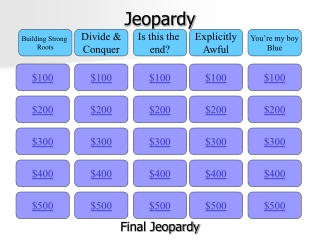DownloadDownload PresentationJeopardy

# Jeopardy

Télécharger la présentation## Jeopardy

- - - - - - - - - - - - - - - - - - - - - - - - - - - E N D - - - - - - - - - - - - - - - - - - - - - - - - - - -
##### Presentation Transcript

1. Jeopardy Building Strong Roots Divide & Conquer Is this the end? Explicitly Awful You’re my boy Blue \$100 \$100 \$100 \$100 \$100 \$200 \$200 \$200 \$200 \$200 \$300 \$300 \$300 \$300 \$300 \$400 \$400 \$400 \$400 \$400 \$500 \$500 \$500 \$500 \$500 Final Jeopardy

2. How many roots would P(x) have (include Real and Imaginary)? P(x) = ax9 + bx8 + cx7 + dx6 + ex5 + fx4 + gx3 + hx2 + jx +k 9 roots Building Strong Roots - \$100

3. Building Strong Roots - \$200 • Completely factor: x2 − 16x + 63 • (x – 9)(x – 7)

4. Building Strong Roots - \$300 • If given the roots 3 and -4 what is the factored form and standard form • (x – 3)(x + 4) x2 + x - 12

5. Building Strong Roots - \$400 • Give all the possible combinations of real and imaginary roots of a cubic function • 3 real, 1 real multiplicity of 3, 1 real 2 imaginary

6. Building Strong Roots - \$500 • Give the factors and roots of f(x) = x4 – 16 • (x – 2)(x + 2)(x – 2i)(x + 2i)

7. Divide and Conquer - \$100 • Given x=2 is a root of the polynomial f(x) = x3 – 3x2 -18x +40, write the polynomial in factored form. • (x – 2)(x + 4)(x + 5)

8. Divide and Conquer - \$200 • Is 2x – 1 a factor of 2x3+15x2-34x+13? • Yes 2x – 1 is a factor

9. Divide and Conquer - \$300 • Give the multiplication statement if you divide x3 – 8x2 – 111x + 10 by x + 7 • (x + 7)(x2 – 15x – 6 + (52 / x+7))

10. Divide and Conquer - \$400 • Given the factor (x – 5) what are the all the linear factors of x3 – 9x2 + 23x - 15 • (x – 1)(x – 3)(x – 5)

11. Divide and Conquer - \$500 • 75x – 40

12. Is this the end? - \$100

13. Is this the end? - \$200 • Is the degree odd or even? And is the leading coefficient positive or negative? • The degree is even and the leading coefficient is positive

14. Is this the end? - \$300

15. Is this the end? - \$400 • Degree is odd and leading coefficient is negative

16. Is this the end? - \$500

17. Explicitly Awful - \$100 • What is the recursive formula of the table? • f(1) = 3; f(n) = f(n – 1) +3

18. Explicitly Awful - \$200 • What is the explicit formula of the table? • f(n) = 3 + 3(n – 1) Or f(n) = 3n

19. Explicitly Awful - \$300 • What is the explicit formula for the table • f(x) = 4 + 2(n – 1) Or f(x) = 2n + 2

20. Explicitly Awful - \$400 • Identify the type of function and explain the common difference • Quadratic 2nd difference is +3

21. Explicitly Awful - \$500 • What is the explicit equation for the table? • f(x) = x2 +3

22. You’re my boy Blue- \$100 • What college did Mrs. Blue graduate from? Even though her family were fans of a rival school. • NC State University

23. You’re my boy Blue- \$200 • What type of dog does Mrs. Blue have and what is his name? • Boxer mix and Boomer

24. You’re my boy Blue - \$300 • What is Ms. Blue’s fiance’s name and where did they meet? • Ben and college

25. You’re my boy Blue - \$400 • Where is Ms. Blue from? (Spelling and pronunciation is how you get credit) • Albemarle

26. You’re my boy Blue - \$500 • What sport is Ms. Blue a professional athlete at? *Hint: she met Shaquille O’Neal because of this sport • Jump rope

27. Final Jeopardy